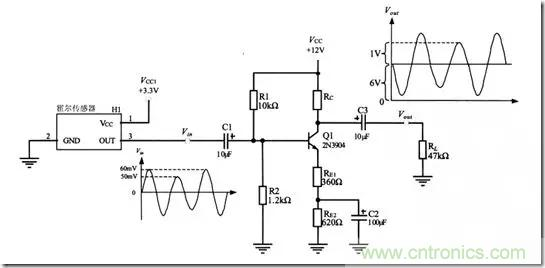## 如何选取共射极放大电路三极管各参数？

【导读】在选取三极管时，需要注意需要了解三极管的几个参数，一个是Vceo，饱和Ic，饱和Vce，集电极极限电流Icm，极限功率Pcm，三极管放大倍数hFE,注意在选取三极管时，集电极输出电流不能超过集电极极限电流Icm，否则会烧坏三极管。

1.Vcq为集电极的静态工作电压，Vcq的选取为了避免出现饱和和截止失真，使Vcq ≈ 1/2 * Vcc，Rc = 10Re；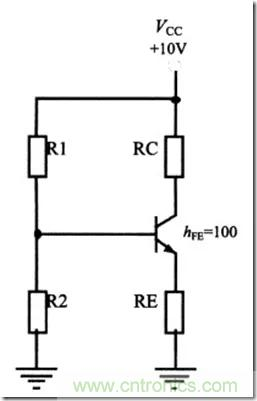Vcq ≈ 1/2 * Vcc = 5V

Icq =（ VCC – Vcq）/Rc = 5mA ；
Icq≈Ieq = 5mA；
Veq = Ieq * Re = 0.5V；

Vbq = Vbe + Veq = 1.2V；

Vbq = R2 /(R1 + R2) * Vcc;

R2 = 1.4KΩ，R1 = 10KΩ，Rc = 1KΩ，Re = 100Ω;

2.交流小信号放大电路（共射极放大电路）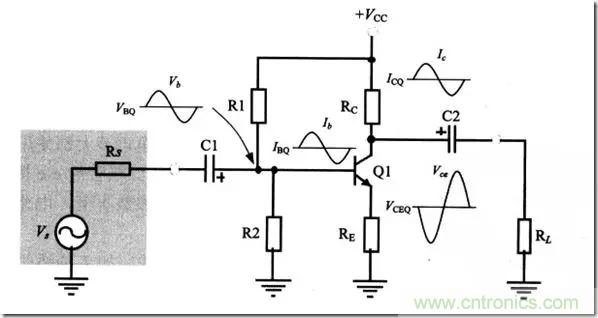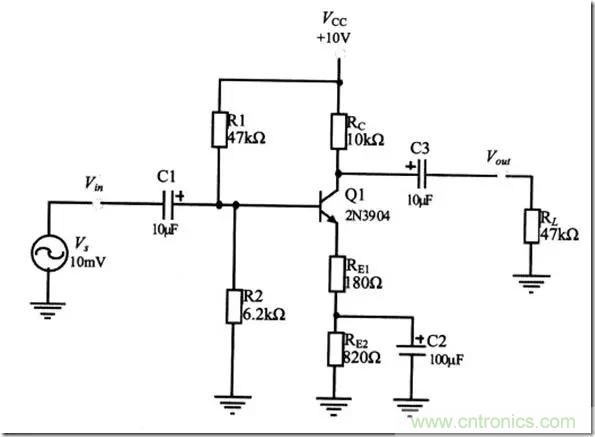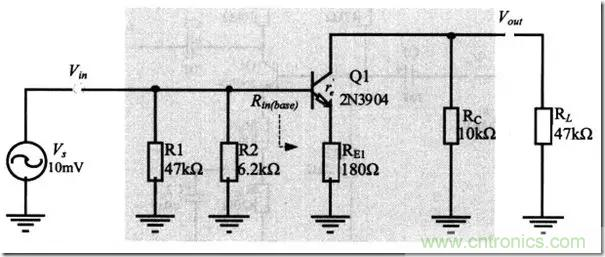Vcq ≈ 1/2 * Vcc，Rc = 10Re；
re’ = 25/Ieq;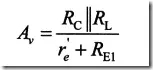（电压增益计算公式）

3.举例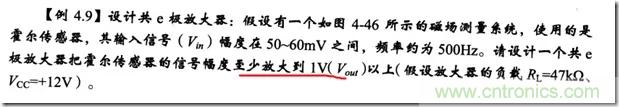Av = 1 / 0.05 = 20;//============================================

Vcq ≈ 1/2 * Vcc = 6V

Icq =（ VCC – Vcq）/Rc = 0.6mA ；
Icq≈Ieq =0.6mA；
//============================================
re’ = 25/Ieq = 42Ω；
Re1 = 370Ω；
Rc = 10Re；
Re1 + Re2 = 1KΩ；
Re2 = 630Ω；
//============================================
Veq = Ieq * （Re1+Re2） = 0.6V；

Vbq = Vbe + Veq = 1.3V；

Vbq = R2 /(R1 + R2) * Vcc;

R2 = 1.2KΩ，R1 = 10KΩ，Rc = 10KΩ，Re1 = 360Ω，Re2 = 640Ω；放大倍数为20；